amortization.com Ltd.
Burlington, Ontario

905-639-0374
905-407-7988

info@amortization.comExcessive Interest Rates

Excessive Interest Rates

QUESTION: If someone charged you \$9.46 cents in interest to borrow \$100 for one week would you be able to calculate the annual interest rate or the effective interest rate the lender was using? Obviously you are able to recognize that \$9.46 is excessive interest. Could you calculate the interest rate and see if it was greater than the Canadian criminal rate of 60%? You could ask your accountant to do the calculation. What if you do not have an accountant. The following example will demonstrate that apparently some lenders do not know exactly what interest rates they are charging, so don’t feel bad. Here is how you can calculate the interest rate charged in that example. Actually there is an upset consumer suing a credit card company for just that reason. The credit card company according to this newspaper article does not appears to know the interest rate they are charging. If you know an accountant or you know how to use a financial calculator then arriving at the answer is easy!

First of all, one must understand the following five definitions before delving into the example. Click here to see the definitions in detail.

Lets do a “PV/FV” calculation on the example from the newspaper article.

Lets not split hairs and fall into the semantics trap. The borrower has to pay back the \$100 plus \$0.46 in “interest” plus \$1.50 as a “transaction fee” plus a “transaction fee interest rate” of \$7.50 for a total of \$109.46

The bottom line, a borrower has borrowed \$100 and has to pay back \$109.46 after 7 days.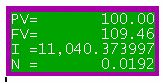Enter PV= \$100
Enter FV = \$109.46
Enter n = 7/365 = 0.019178 years
Calculate the I% = 11,040.37% (that’s eleven thousand percent)

The calculated interest rate of 11,040% using the PV/FV calculator is actually the effective interest rate, EIR. In mortgage broker terminology in the province of Ontario its called the Total Cost of Borrowing. The nominal interest rate using daily compounding (worse case scenario) could be arrived at as follows (this is the simplest way instead of resorting to equations). Using the MORTGAGE2 PRO calculator let us assume we will borrow \$100 and pay weekly payments over an amortization period of one year. We type in bigger and bigger annual interest rates (AIR) until we see that the interest portion of the very first weekly payment, in the amortization schedule is \$9.46 When this happens the AIR or the nominal annual interest rate is 474.42% which translates into an EIR of 11,040%

The statement from the Bank reads the annual interest rate is 94.11%. This does not agree with either the 474% or 11,040%. This type of problem could easily be AVOIDED if all the provincial governments and the federal government were on the same page and used the same financial definitions and terminology.

(Screenshot 1)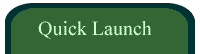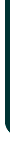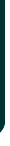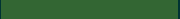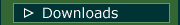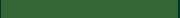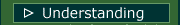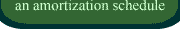VIDEOSamortizationdotcom Mortgage Calculator for iPhone

Introduction to Canadian and American Mortgages

Seminar on prepaying principal (Part A)

Seminar on prepaying principal (Part B)

Global TV Interview regarding 40 Year MortgagesLook for this logo on the Apple Store!

 < Go Back